# 留存分析

• 文档创建者：axing
• 编辑次数：2次
• 最近更新：Carly 于 2021-02-01
• ## 1. 概述

### 1.1 概念

留存分析是一种用来分析用户参与情况/活跃程度的分析模型，考查看进行初始行为后的用户中， 经过一段时间后仍然存在客户行为（如登录、消费）。

留存不仅是个可以反映客户粘性的指标，更多地反映产品对用户的吸引力。

计算公式：某一段时间内（时间段a）的新增用户在若干天后的另一段时间（时间段b）的留存数量 / （时间段a）的新增用户总量

### 1.2 预期效果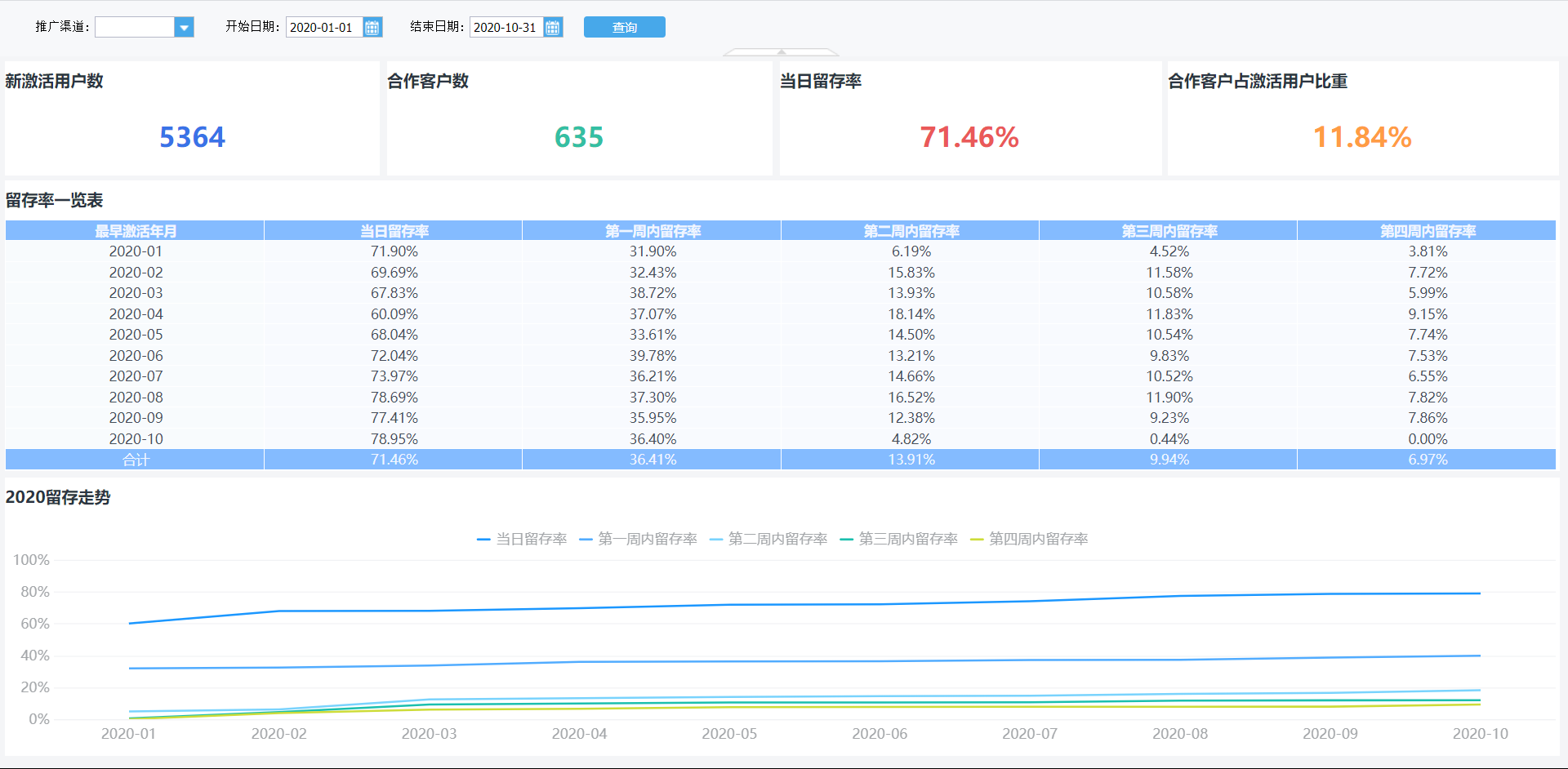### 1.3 实现思路

示例计算用户从激活某产品开始，在当日、一周内、两周内、三周内进行登录使用操作用户数占总登录人数的比率。

当日留存率：当日激活并登录用户数/所有登录用户数

一周留存率：初次激活日为维度，计算「激活_登录时间差」为 1-7 的登录用户数/所有登录用户数

两周留存率：初次激活日为维度，计算「激活_登录时间差」为8-14的登录用户数/所有登录用户数

.....

## 2. 示例

### 2.1 导入数据

示例数据：

将数据表上传至 FineReport 内置数据库 FRDemo 中。

### 2.2 新建模板

新建决策报表，新建数据集查询 ds1：select * from 用户留存分析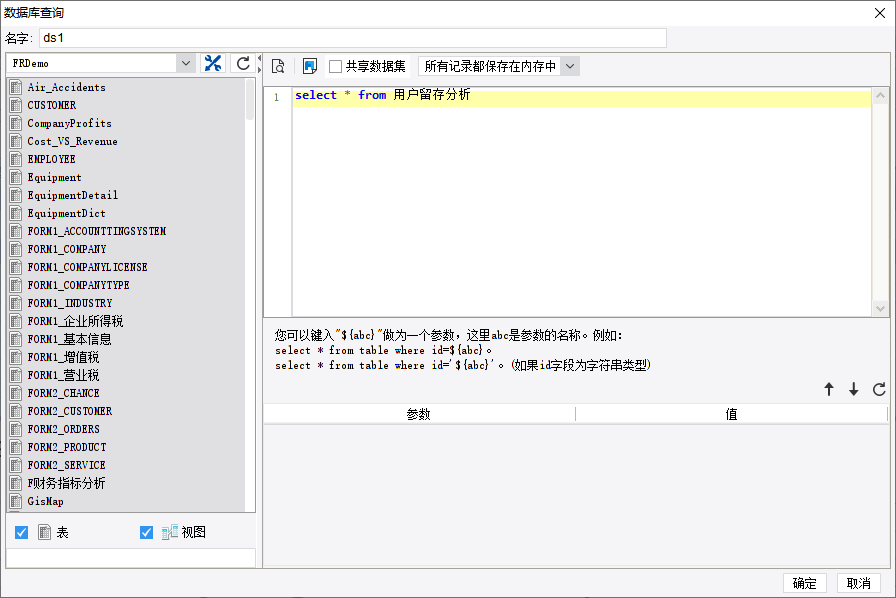### 2.3 单元格设计

1）新建报表块，单元格设计如下图所示：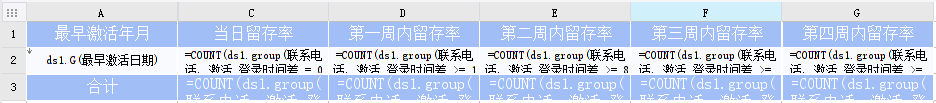2）选中 A2 单元格，设置分组-高级，自定义公式分组，公式为：left(\$\$\$,7)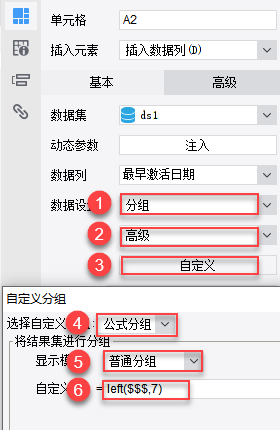3）其余单元格设计如下：

单元格
公式
C2COUNT(ds1.group(联系电话, 激活_登录时间差 = 0 && left(最早激活日期, 7) = \$A2 && 最早激活日期 >= \$开始日期 && 最早激活日期 <= \$结束日期 && if(len(\$渠道) = 0, true, inarray(推广渠道, \$渠道) > 0), true))/B2
D2COUNT(ds1.group(联系电话, 激活_登录时间差 >= 1 && 激活_登录时间差 <= 7 && left(最早激活日期, 7) = \$A2&& 最早激活日期 >= \$开始日期 && 最早激活日期 <= \$结束日期 && if(len(\$渠道) = 0, true, inarray(推广渠道, \$渠道) > 0), true)) / B2
E2COUNT(ds1.group(联系电话, 激活_登录时间差 >= 8 && 激活_登录时间差 <= 14 && left(最早激活日期, 7) = \$A2&& 最早激活日期 >= \$开始日期 && 最早激活日期 <= \$结束日期 && if(len(\$渠道) = 0, true, inarray(推广渠道, \$渠道) > 0), true)) / B2
F2COUNT(ds1.group(联系电话, 激活_登录时间差 >= 15 && 激活_登录时间差 <= 21 && left(最早激活日期, 7) = \$A2&& 最早激活日期 >= \$开始日期 && 最早激活日期 <= \$结束日期 && if(len(\$渠道) = 0, true, inarray(推广渠道, \$渠道) > 0), true)) /B2
G2COUNT(ds1.group(联系电话, 激活_登录时间差 >= 22 && 激活_登录时间差 <= 28 && left(最早激活日期, 7) = \$A2&& 最早激活日期 >= \$开始日期 && 最早激活日期 <= \$结束日期 && if(len(\$渠道) = 0, true, inarray(推广渠道, \$渠道) > 0), true)) /B2
C3COUNT(ds1.group(联系电话, 激活_登录时间差 = 0 && 最早激活日期 >= \$开始日期 && 最早激活日期 <= \$结束日期 && if(len(\$渠道) = 0, true, inarray(推广渠道, \$渠道) > 0), true))/B3
D3COUNT(ds1.group(联系电话, 激活_登录时间差 >= 1 && 激活_登录时间差 <= 7 && 最早激活日期 >= \$开始日期 && 最早激活日期 <= \$结束日期 && if(len(\$渠道) = 0, true, inarray(推广渠道, \$渠道) > 0), true)) / B3
E3COUNT(ds1.group(联系电话, 激活_登录时间差 >= 8 && 激活_登录时间差 <= 14 && 最早激活日期 >= \$开始日期 && 最早激活日期 <= \$结束日期 && if(len(\$渠道) = 0, true, inarray(推广渠道, \$渠道) > 0), true)) / B3
F3COUNT(ds1.group(联系电话, 激活_登录时间差 >= 15 && 激活_登录时间差 <= 21 && 最早激活日期 >= \$开始日期 && 最早激活日期 <= \$结束日期 && if(len(\$渠道) = 0, true, inarray(推广渠道, \$渠道) > 0), true)) /B3
G3COUNT(ds1.group(联系电话, 激活_登录时间差 >= 22 && 激活_登录时间差 <= 28 && 最早激活日期 >= \$开始日期 && 最早激活日期 <= \$结束日期 && if(len(\$渠道) = 0, true, inarray(推广渠道, \$渠道) > 0), true)) /B3

### 2.4 效果预览

PC 端预览效果如 1.2 节所示。

移动端预览效果如下图所示：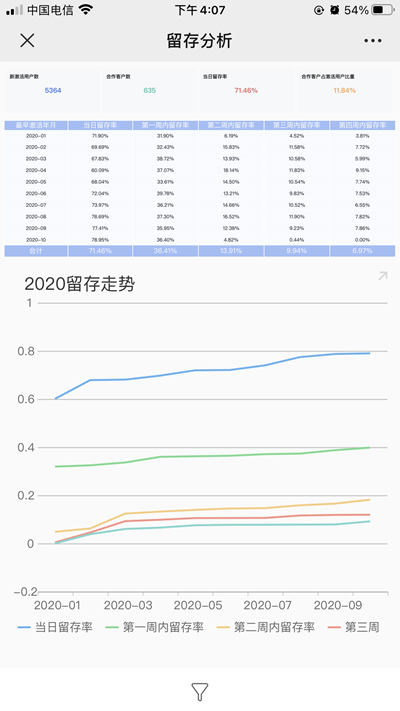## 3. 模板下载

点击下载模板：

• 有帮助
• 没帮助
• 只是浏览
• 评价文档，奖励 1 ~ 100 随机 F 豆！

售前咨询电话

400-811-8890转1

在线技术支持

在线QQ：800049425

热线电话：400-811-8890转2

总裁办24H投诉

热线电话：186-0252-2339